# Intermediate Maths 1B solutions for Applications of Derivatives exercise 10(b) solutions

Applications of Derivatives exercise 10(b) Maths 1B textbook Inter solutions are given.

Study the textbook lesson Applications of Derivatives very well.

Observe the example problems and solutions given in the textbook.

Observe the given below solutions and try them in your own method.

You can also see

Inter Maths 1A textbook solutions

Inter Maths 1B textbook solutions

Inter Maths IIA textbook solutions

Inter Maths IIB textbook solutions

Applications of Derivatives

Exercise 10(a)

Exercise 10(b)

Exercise 10(c)

Exercise 10(d)

Exercise 10(e)

Exercise 10(f)

Exercise 10(g)

Exercise 10(h)

M

## Chapter 10 Applications of Derivatives exercise 10(b) solutions

Applications of Derivatives

Chapter 10 – Exercise 10(b)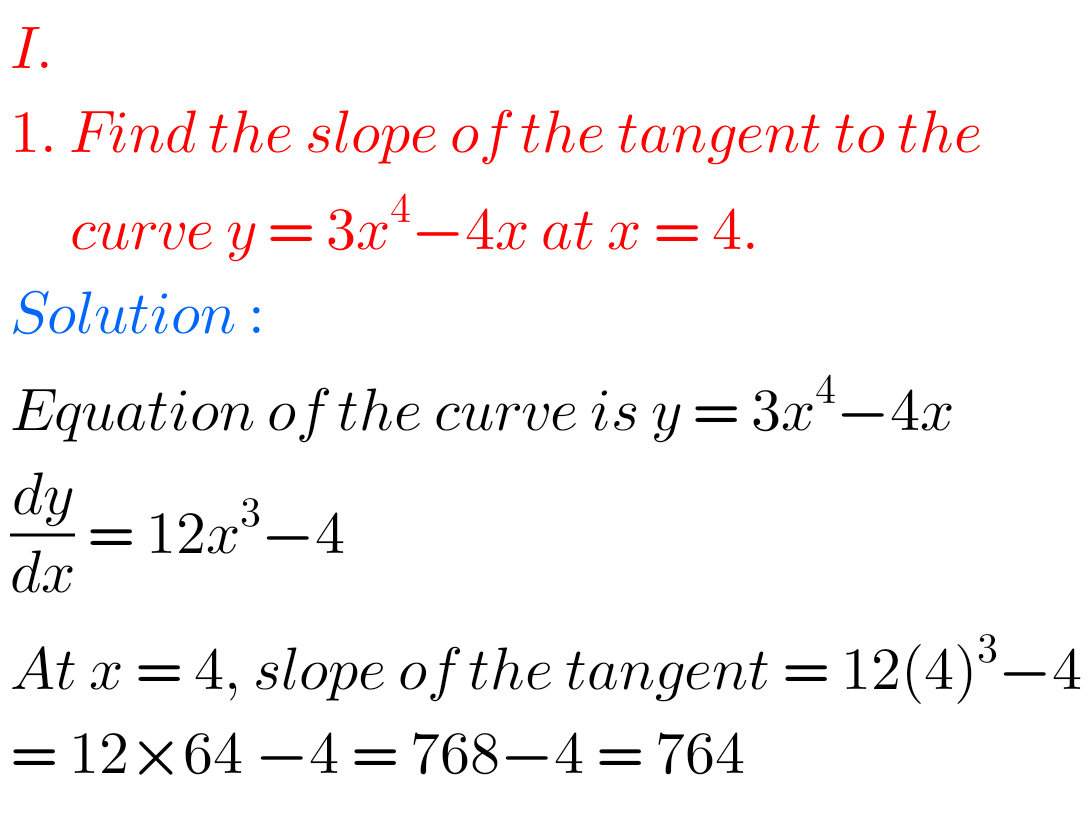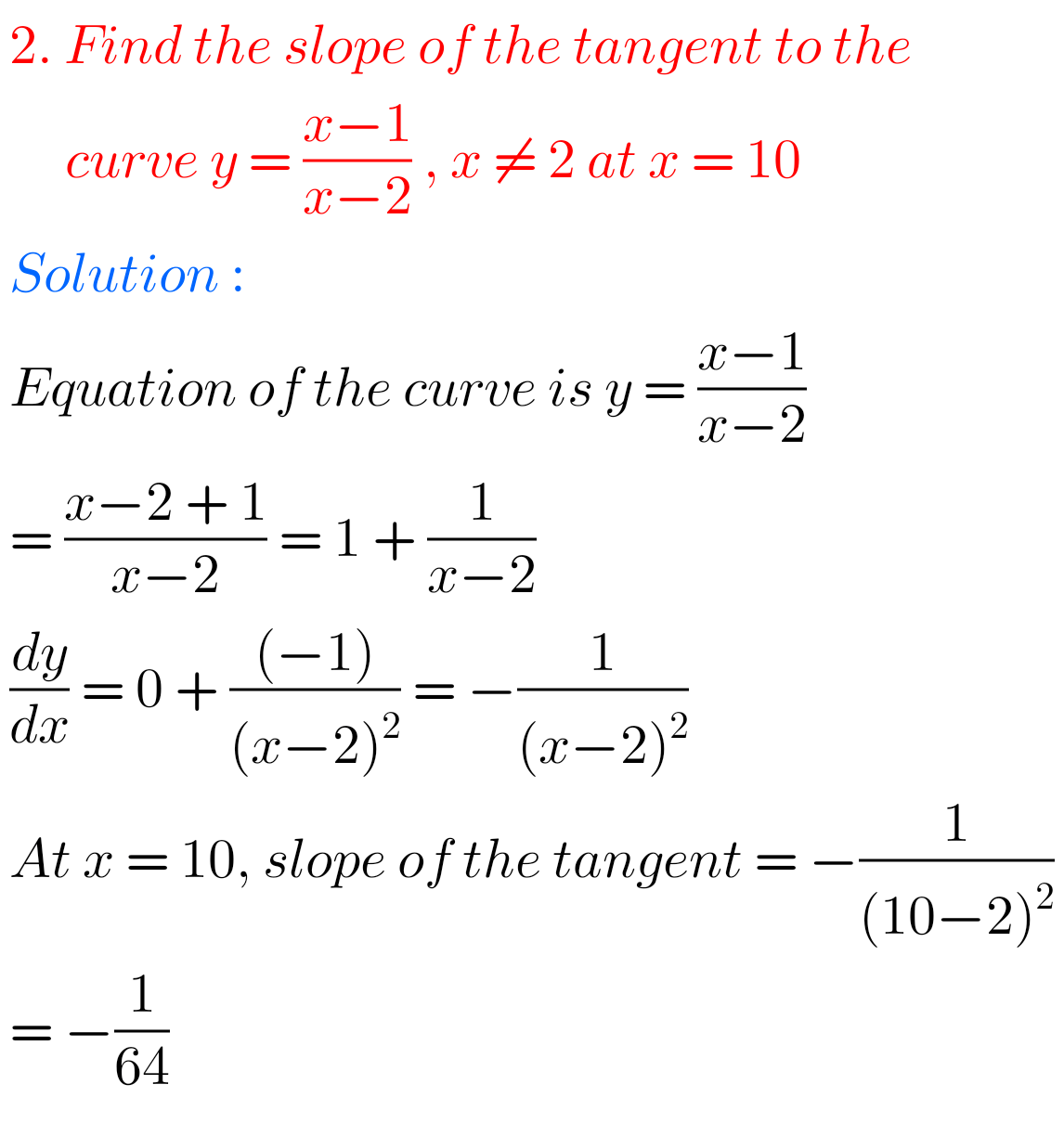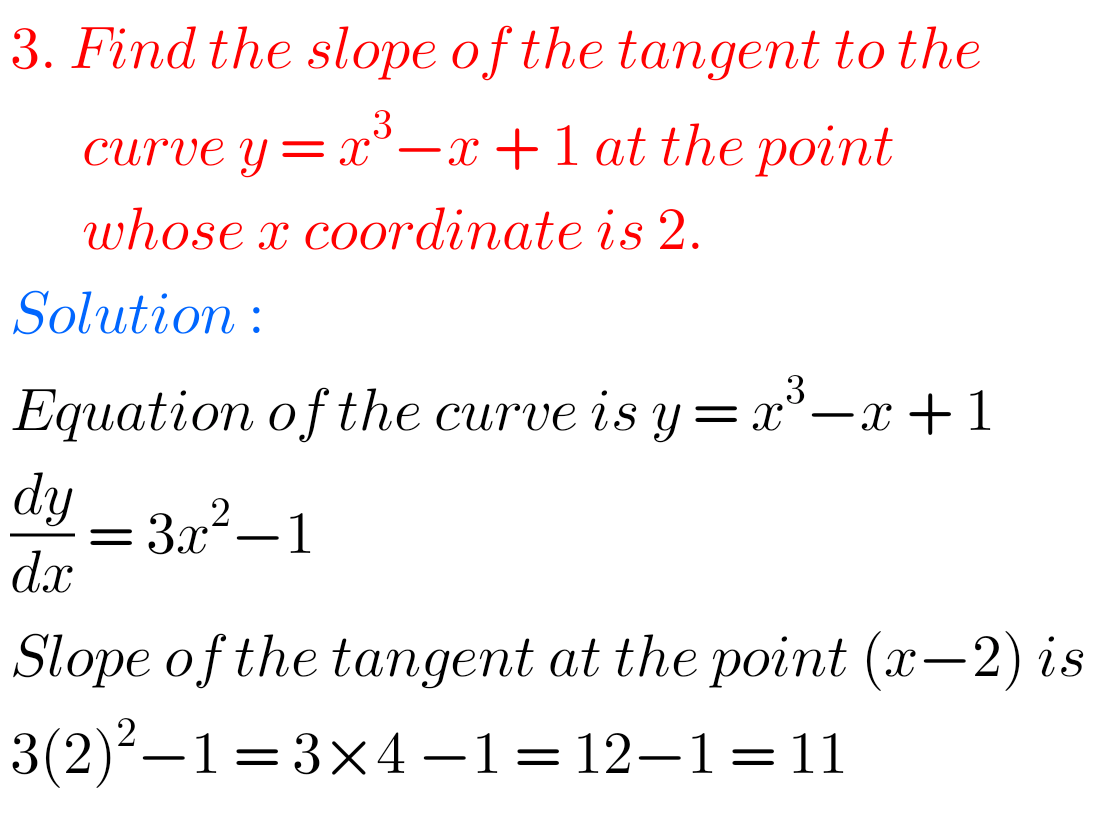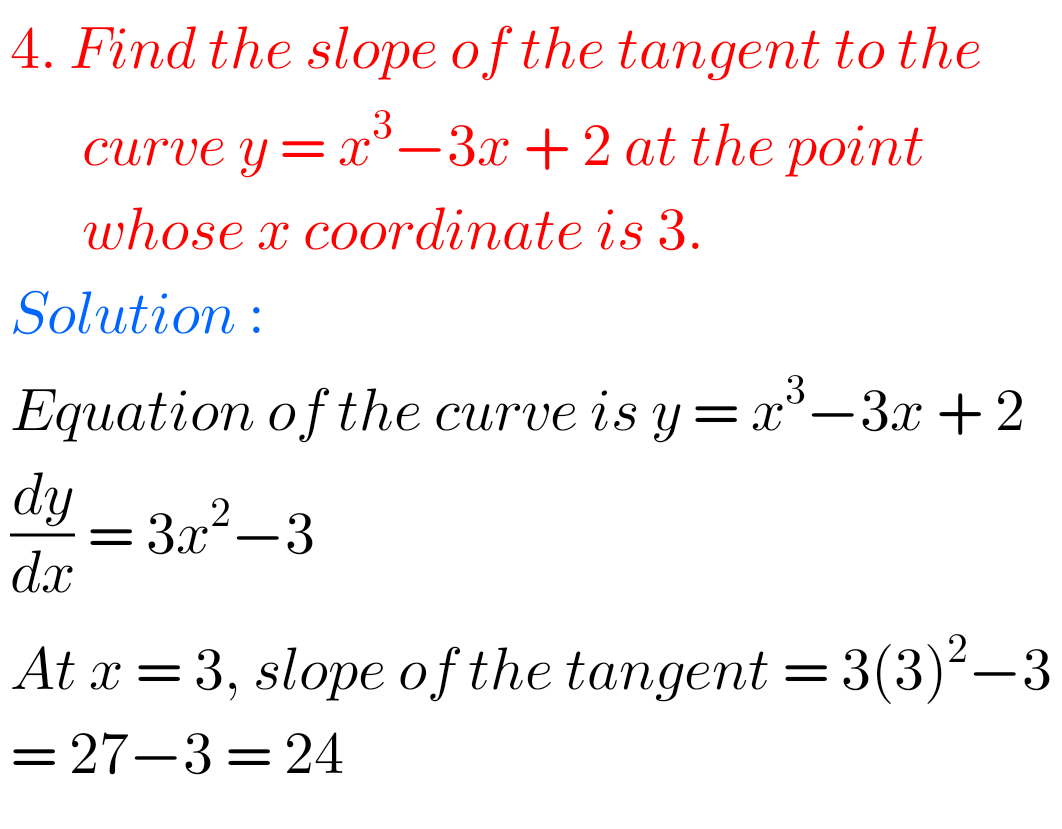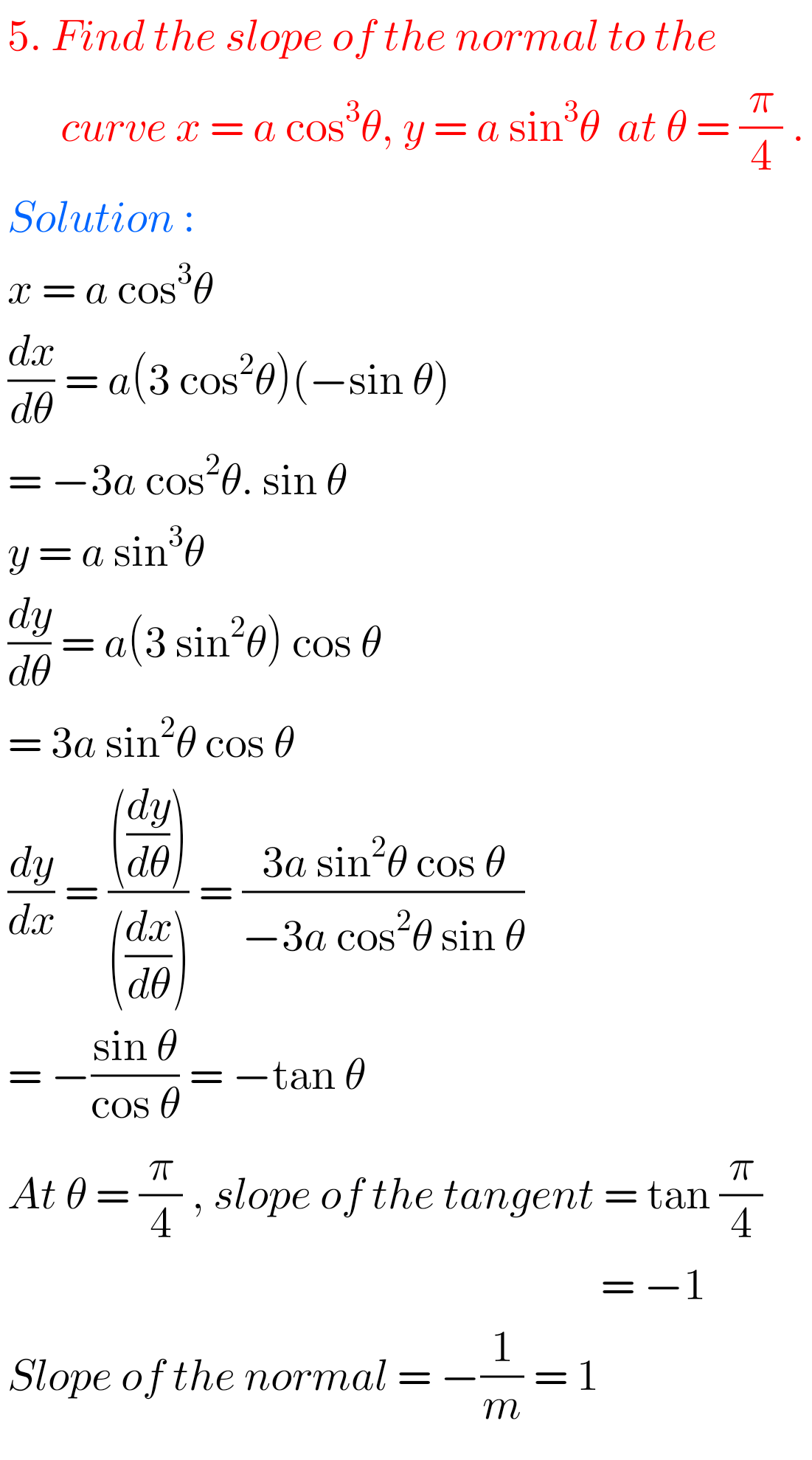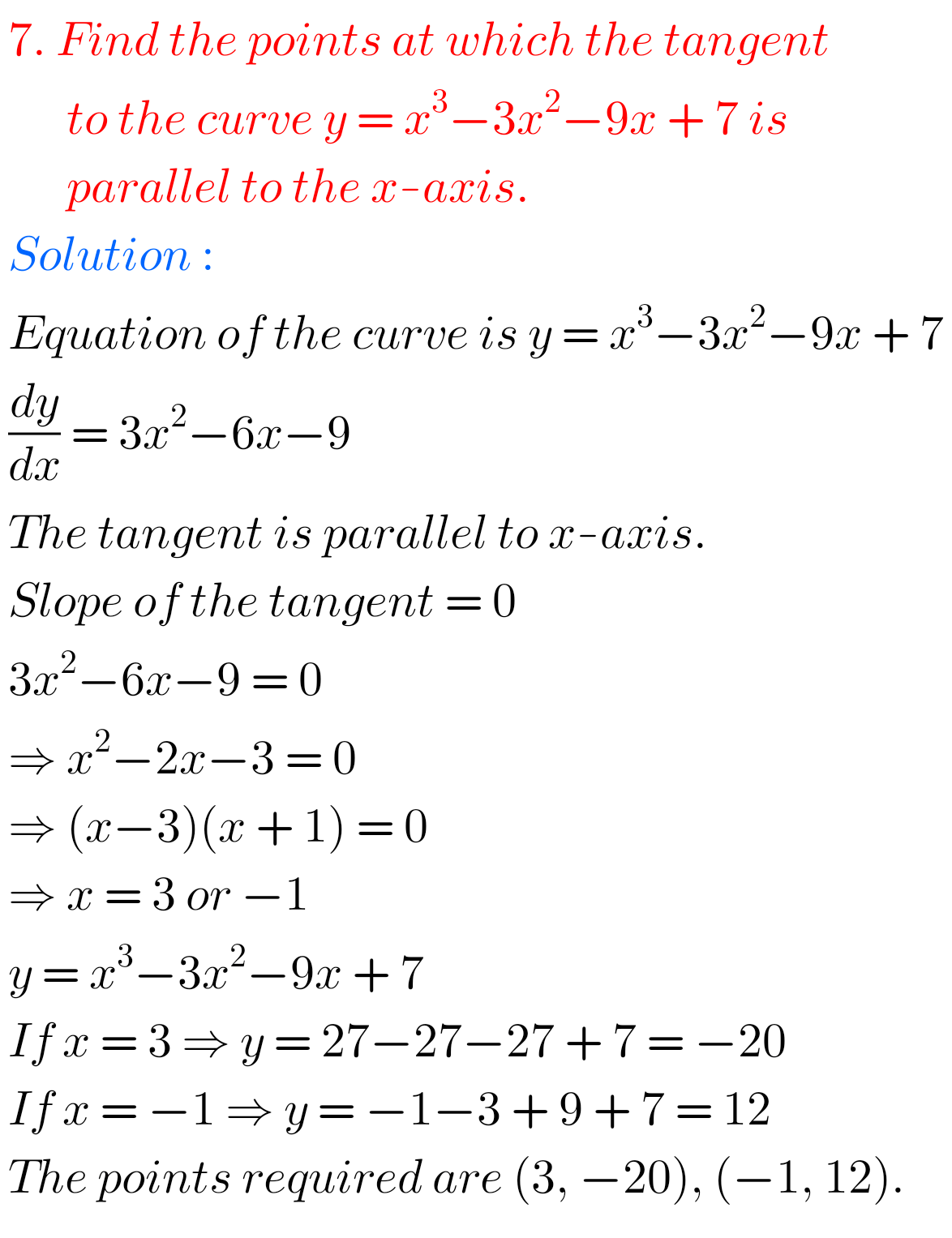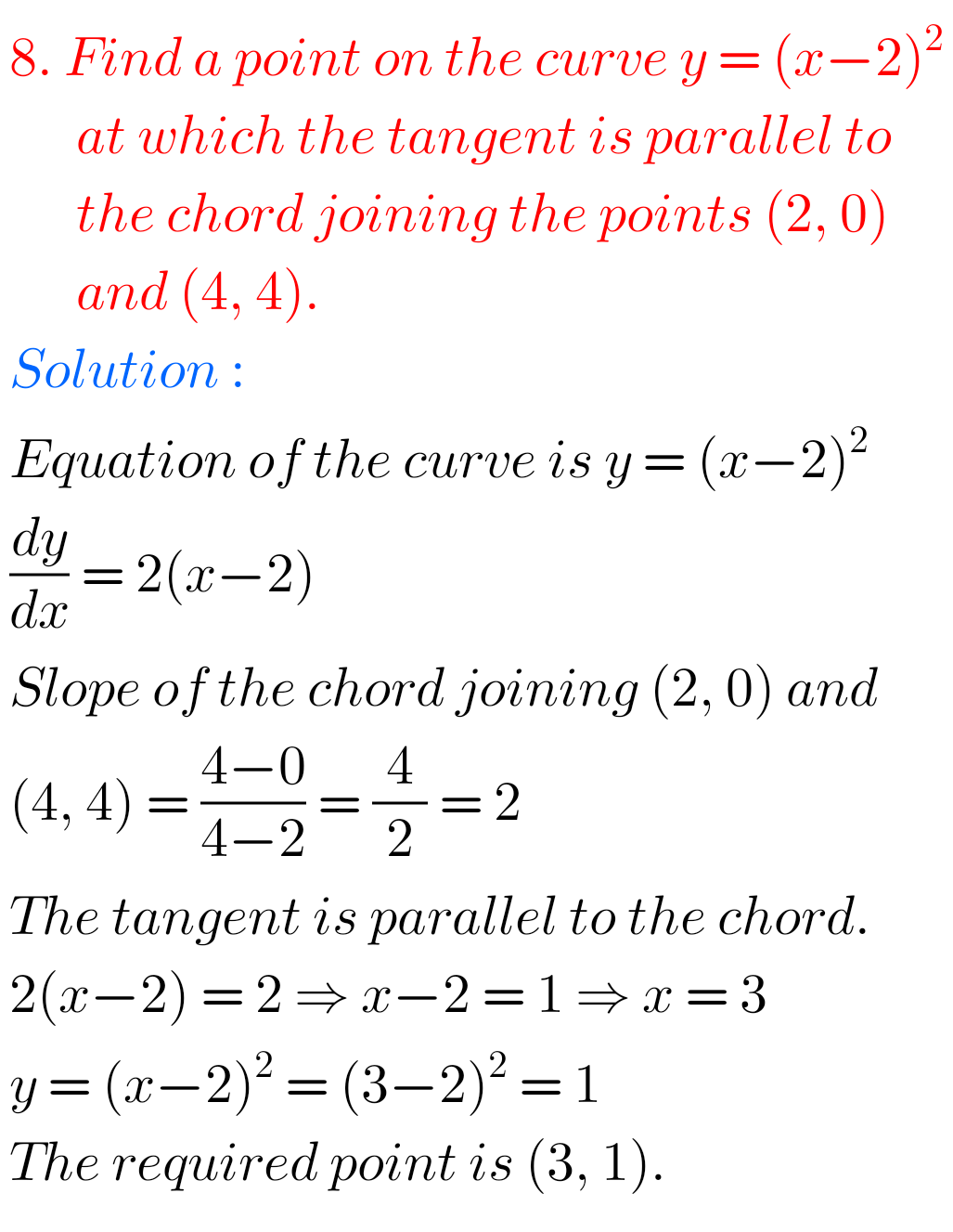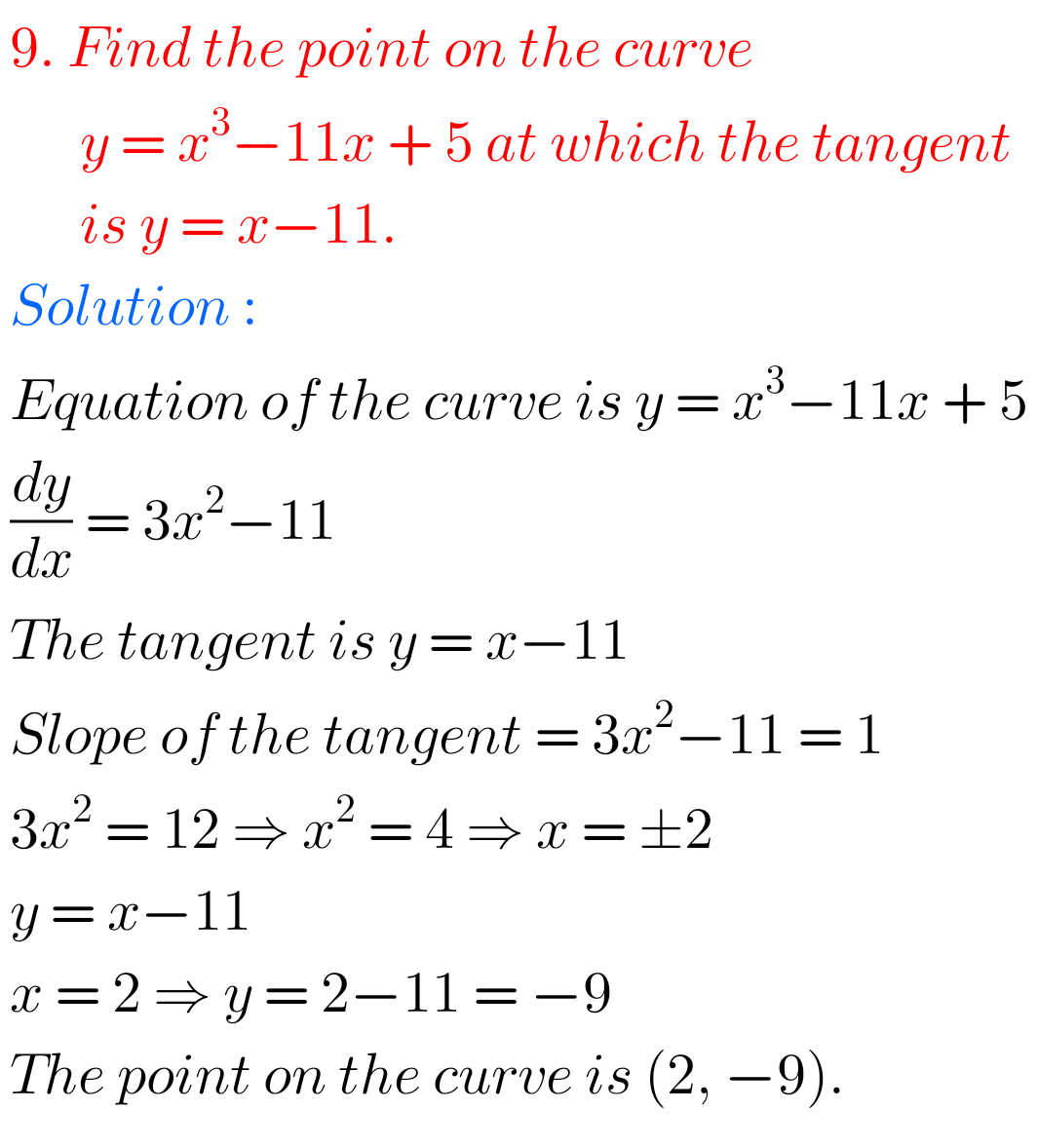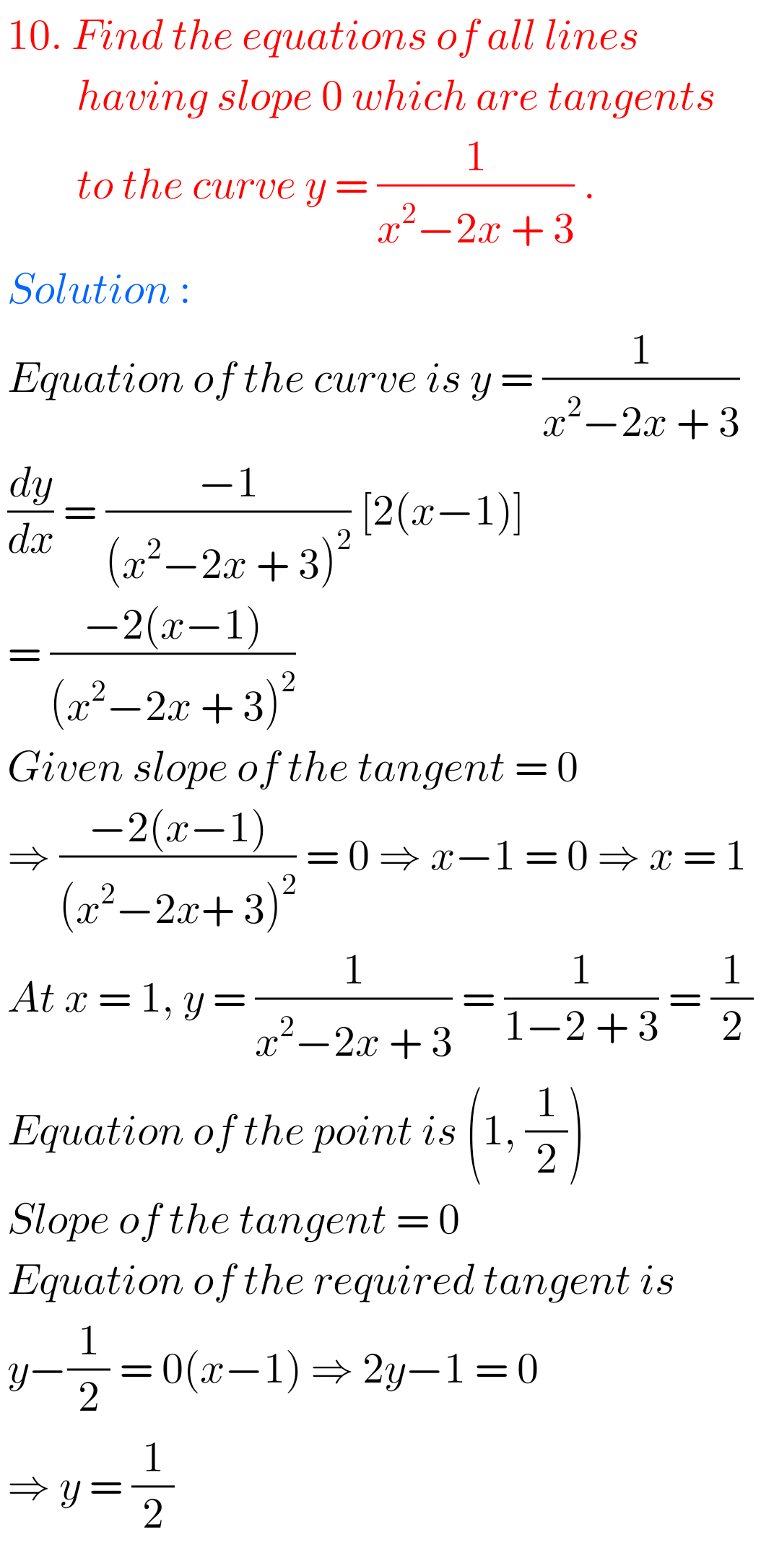## Exercise 10(b) Applications of Derivatives solutions Inter maths 1B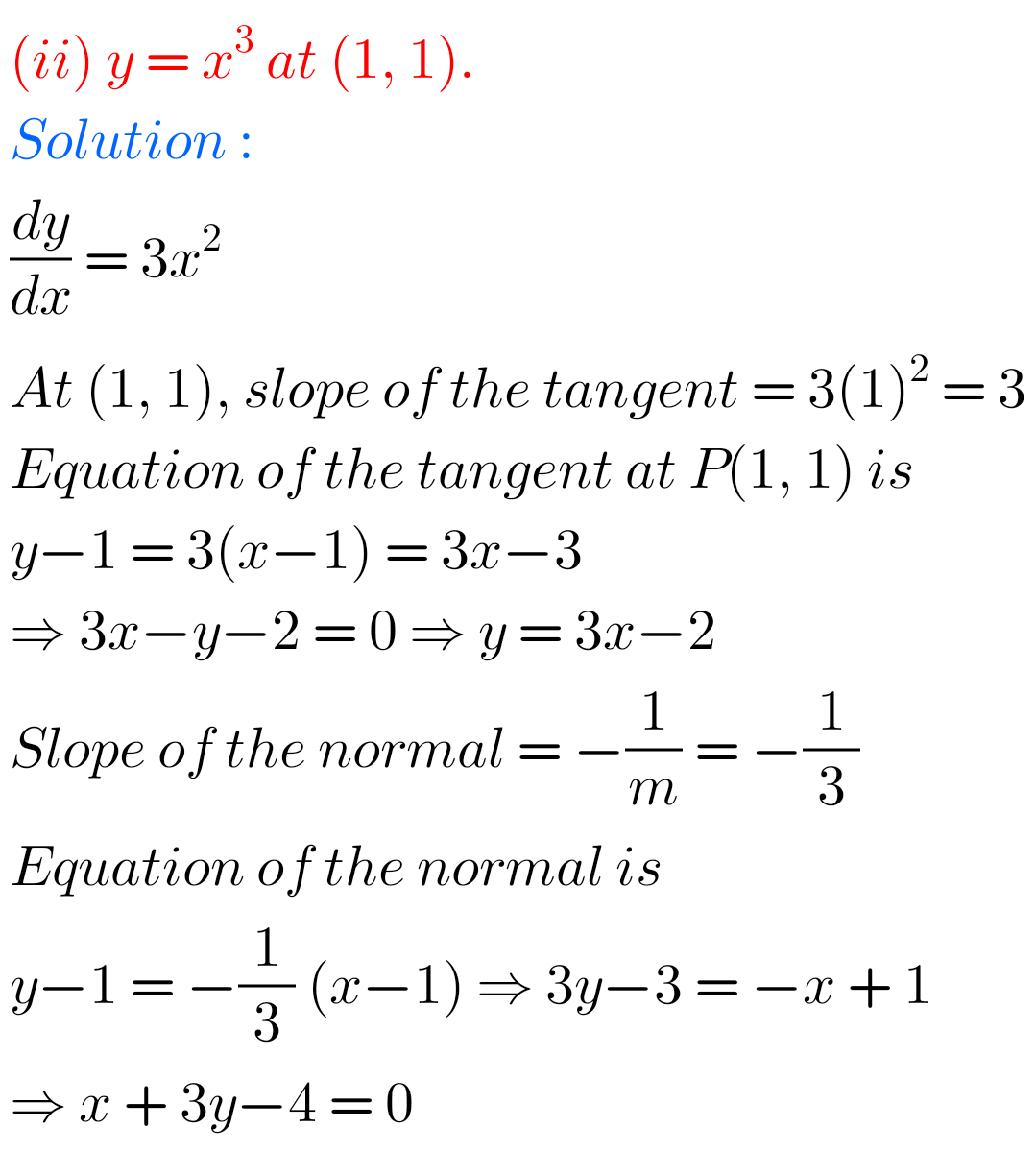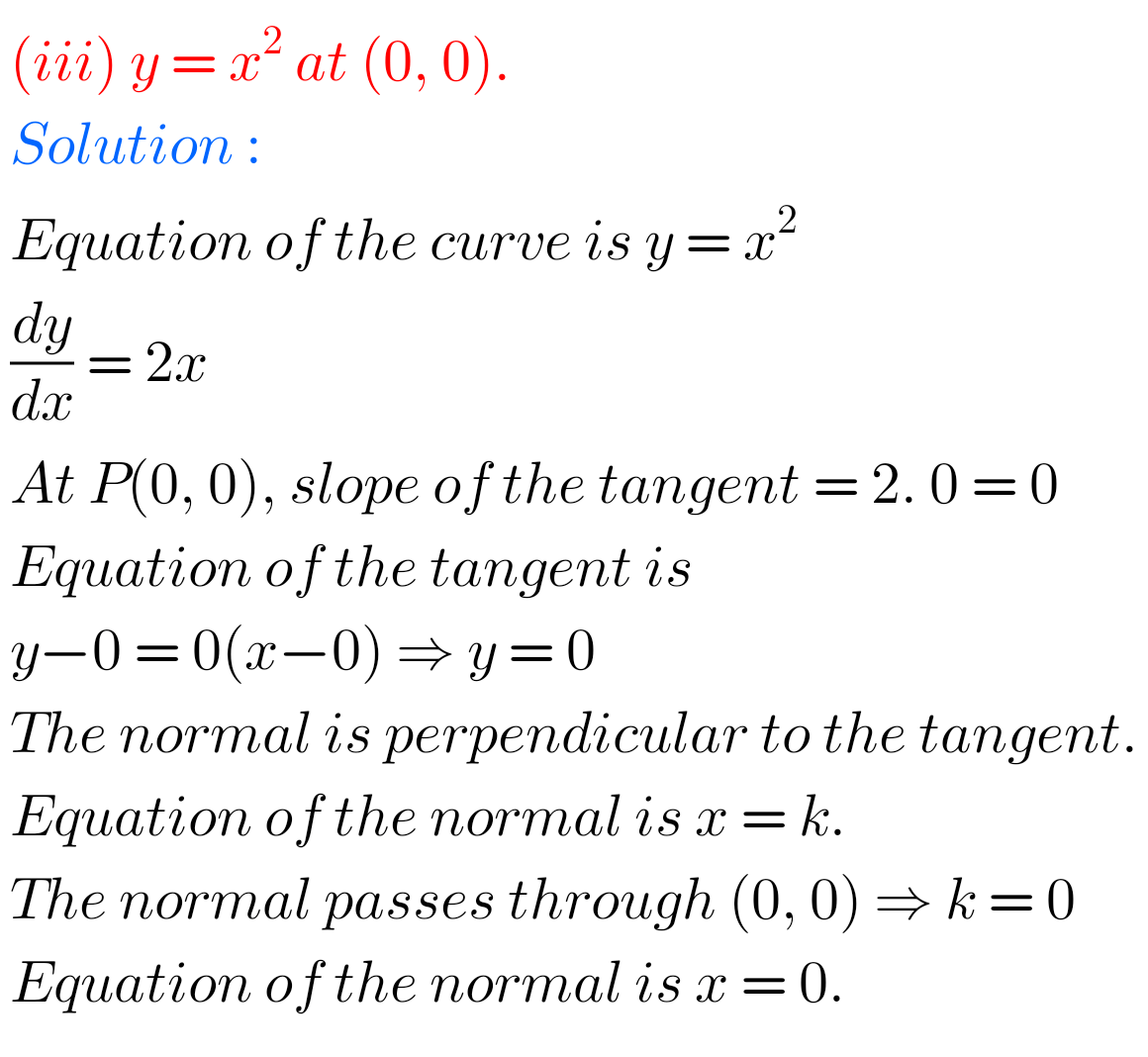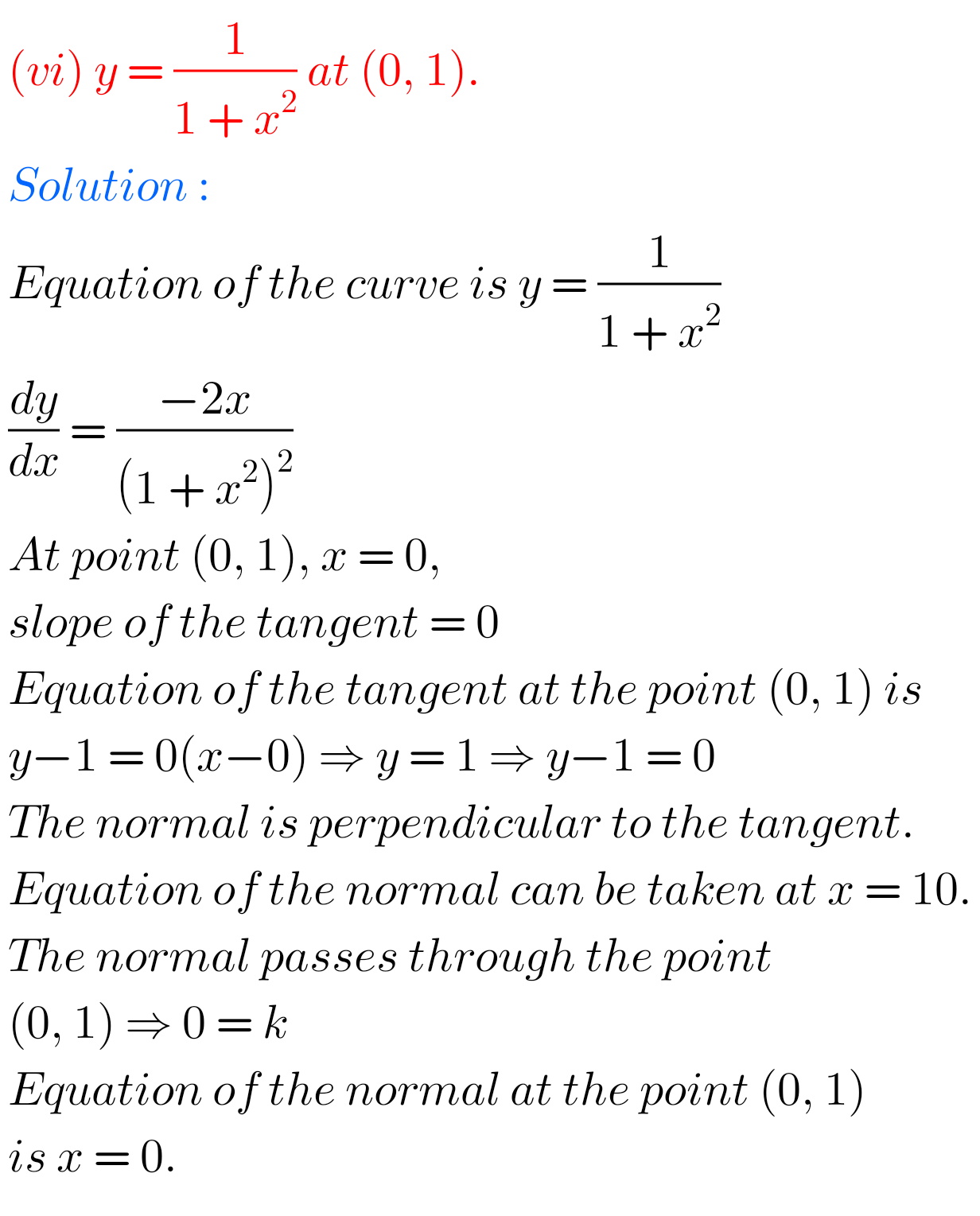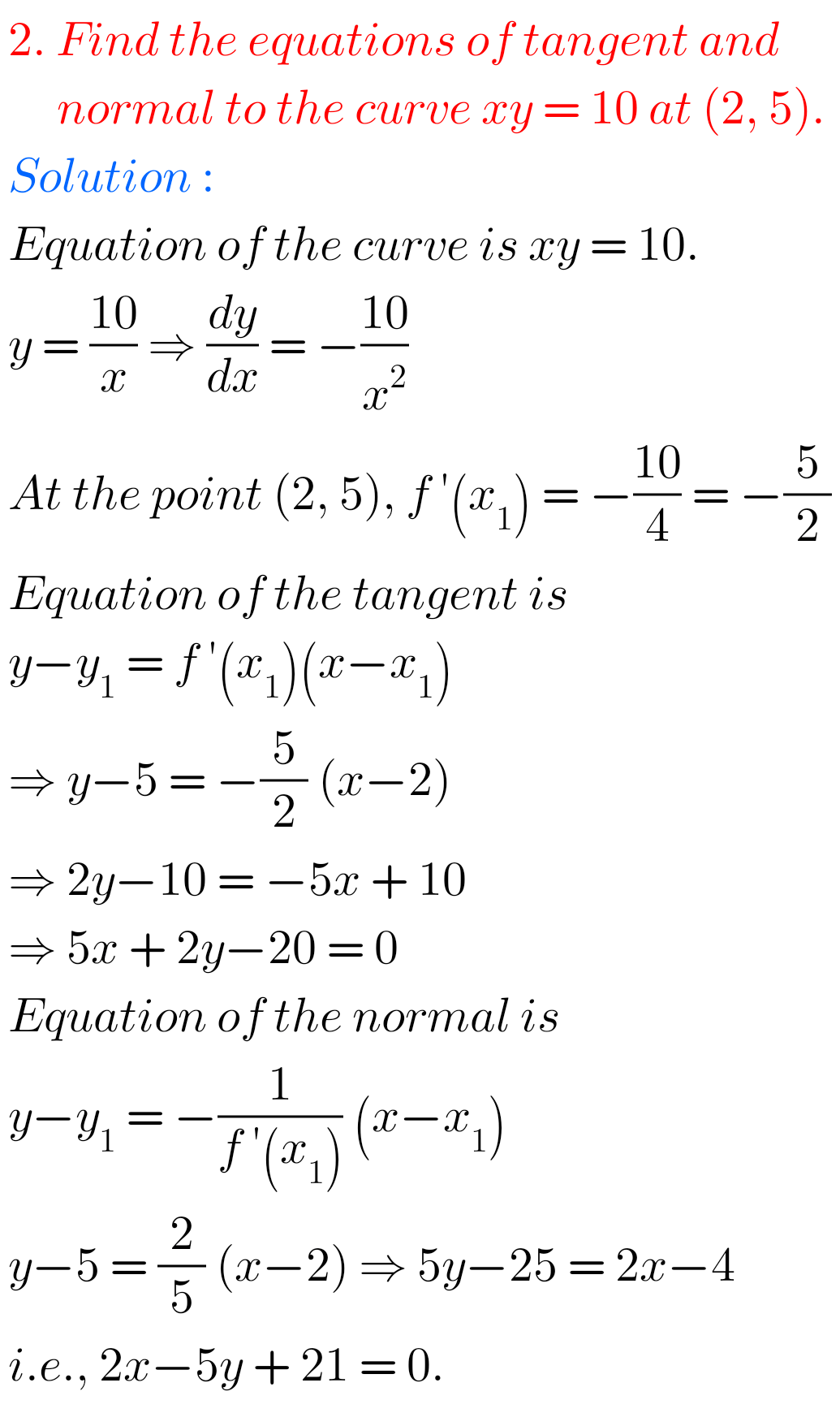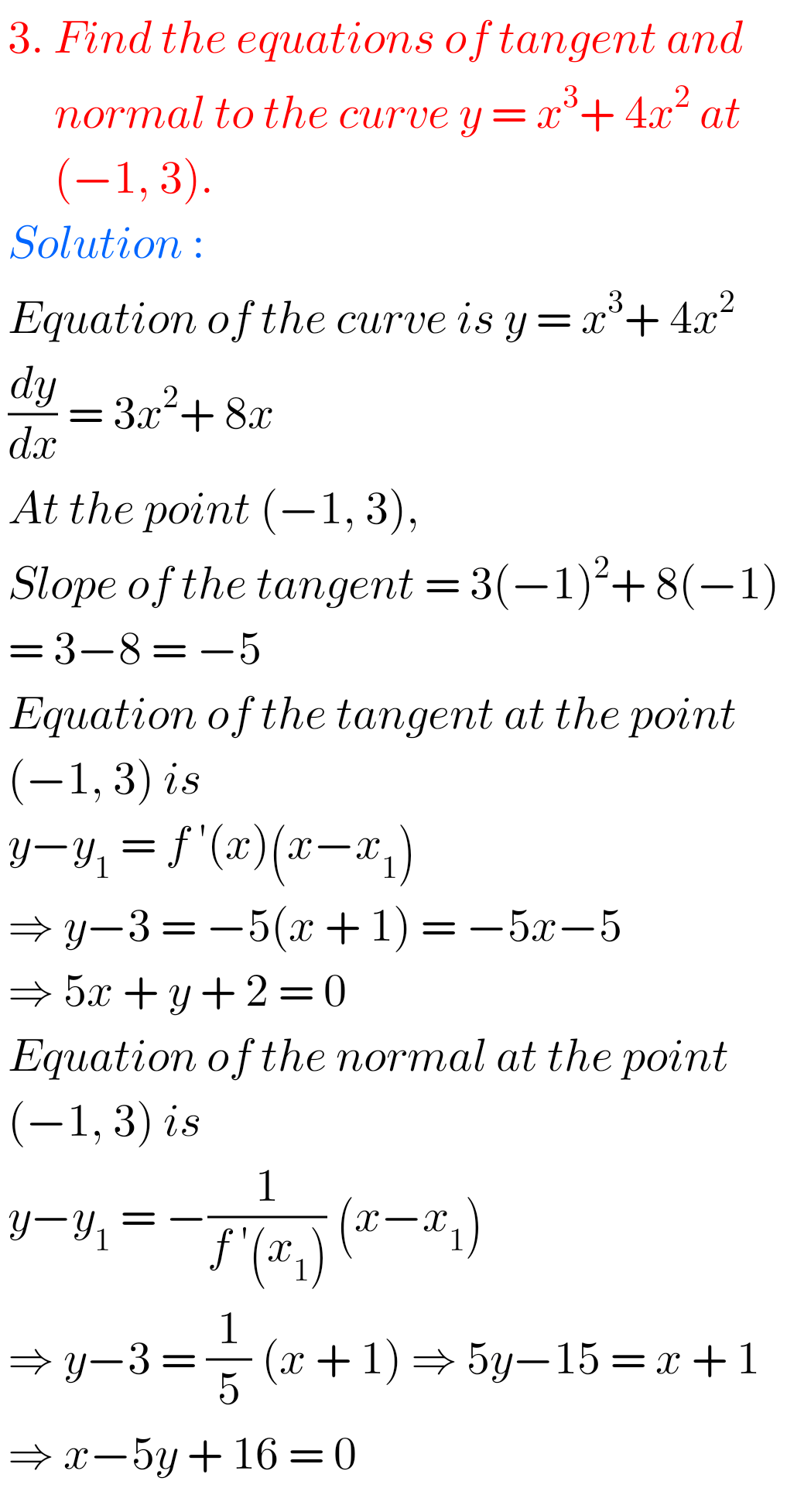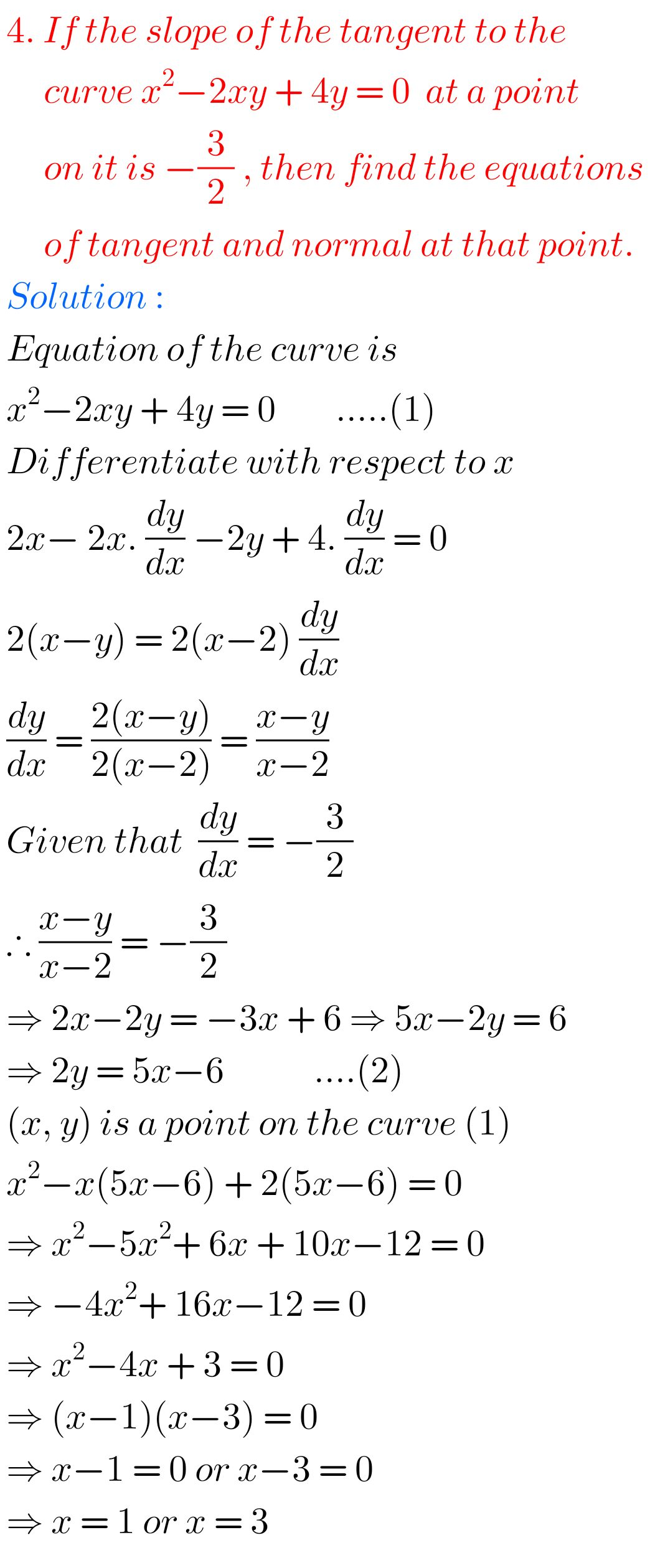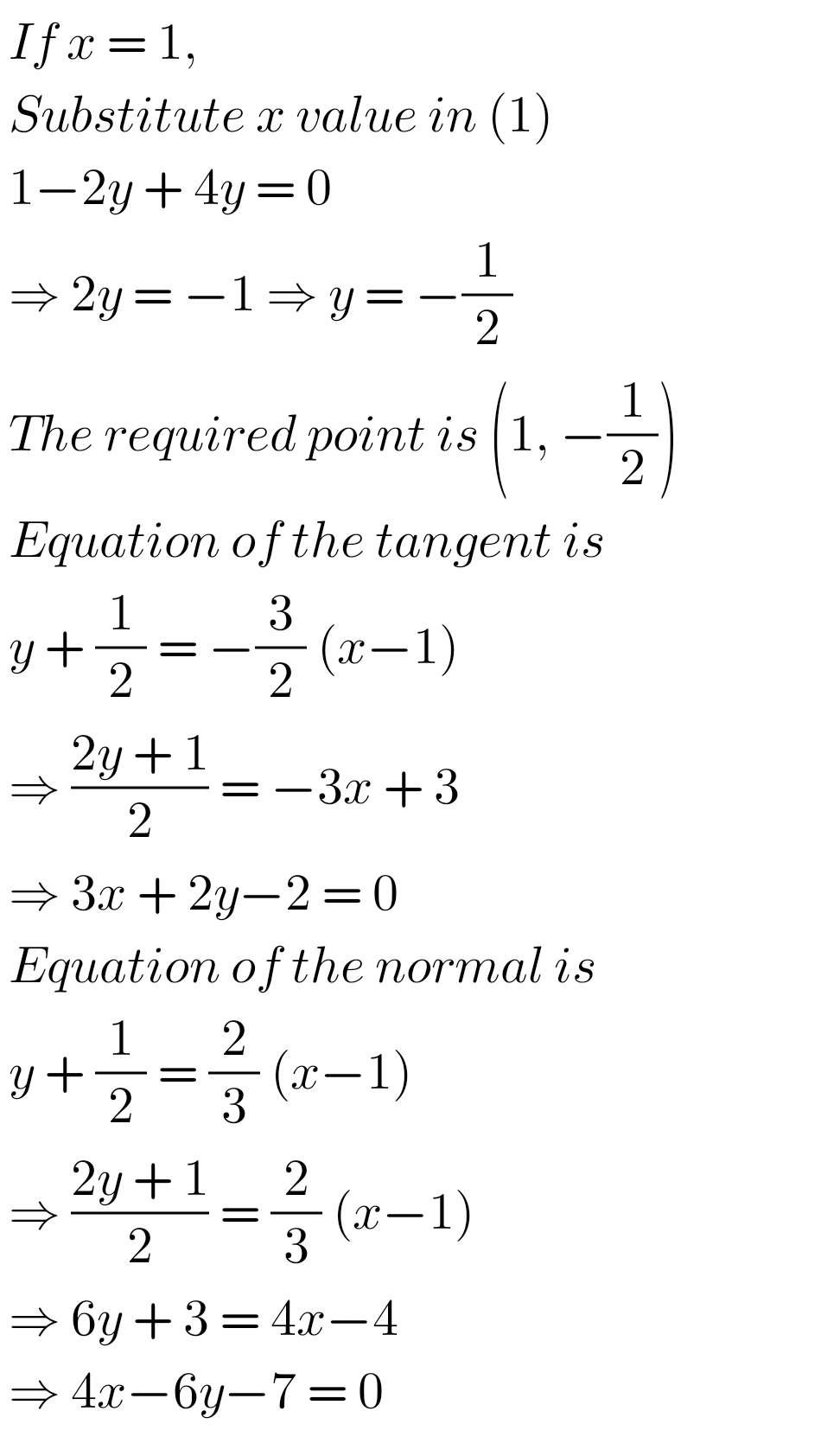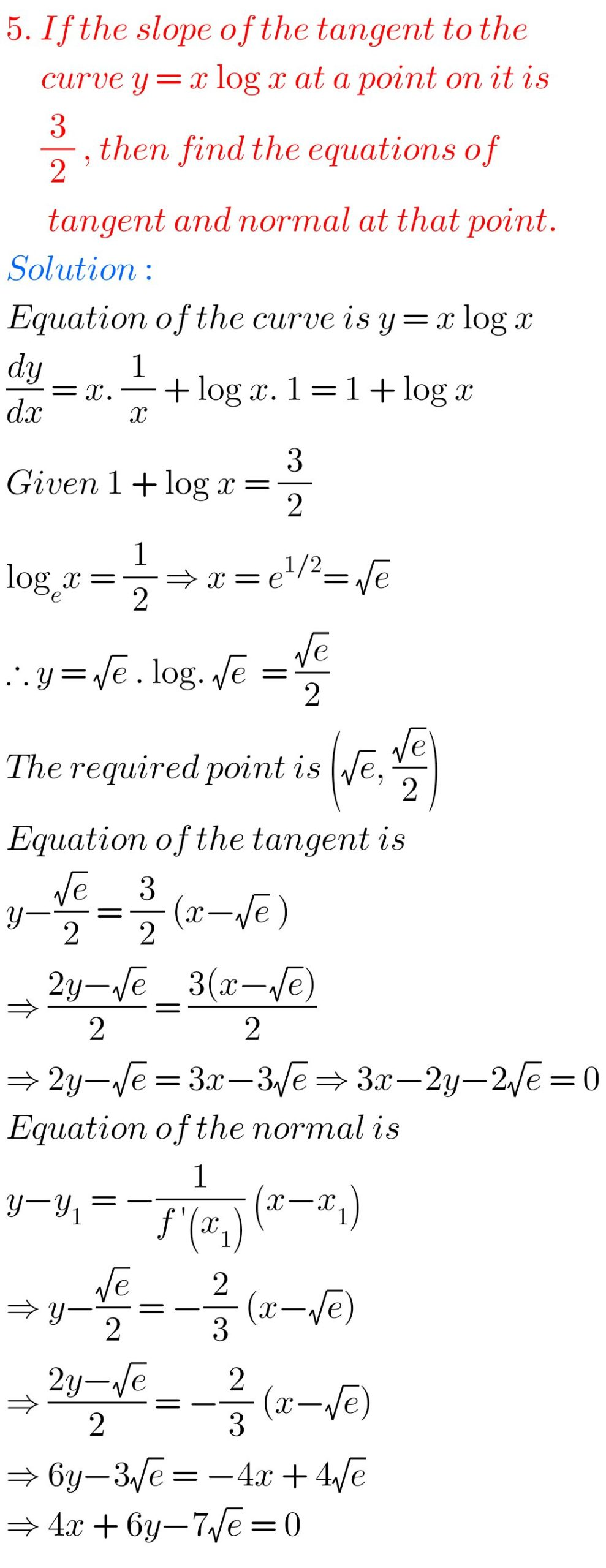## Maths 1B solutions for Applications of Derivatives Exercise 10(b) solutions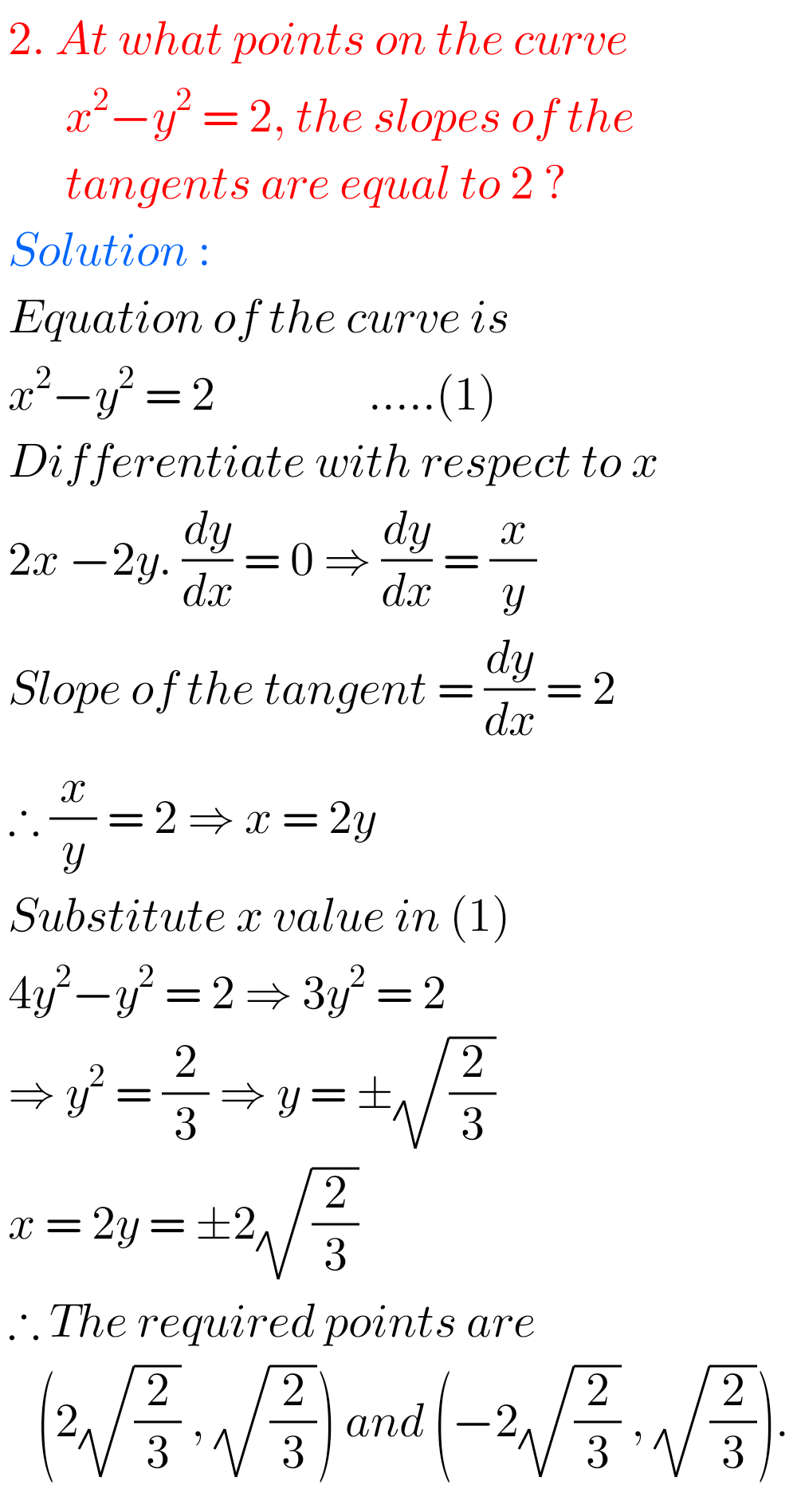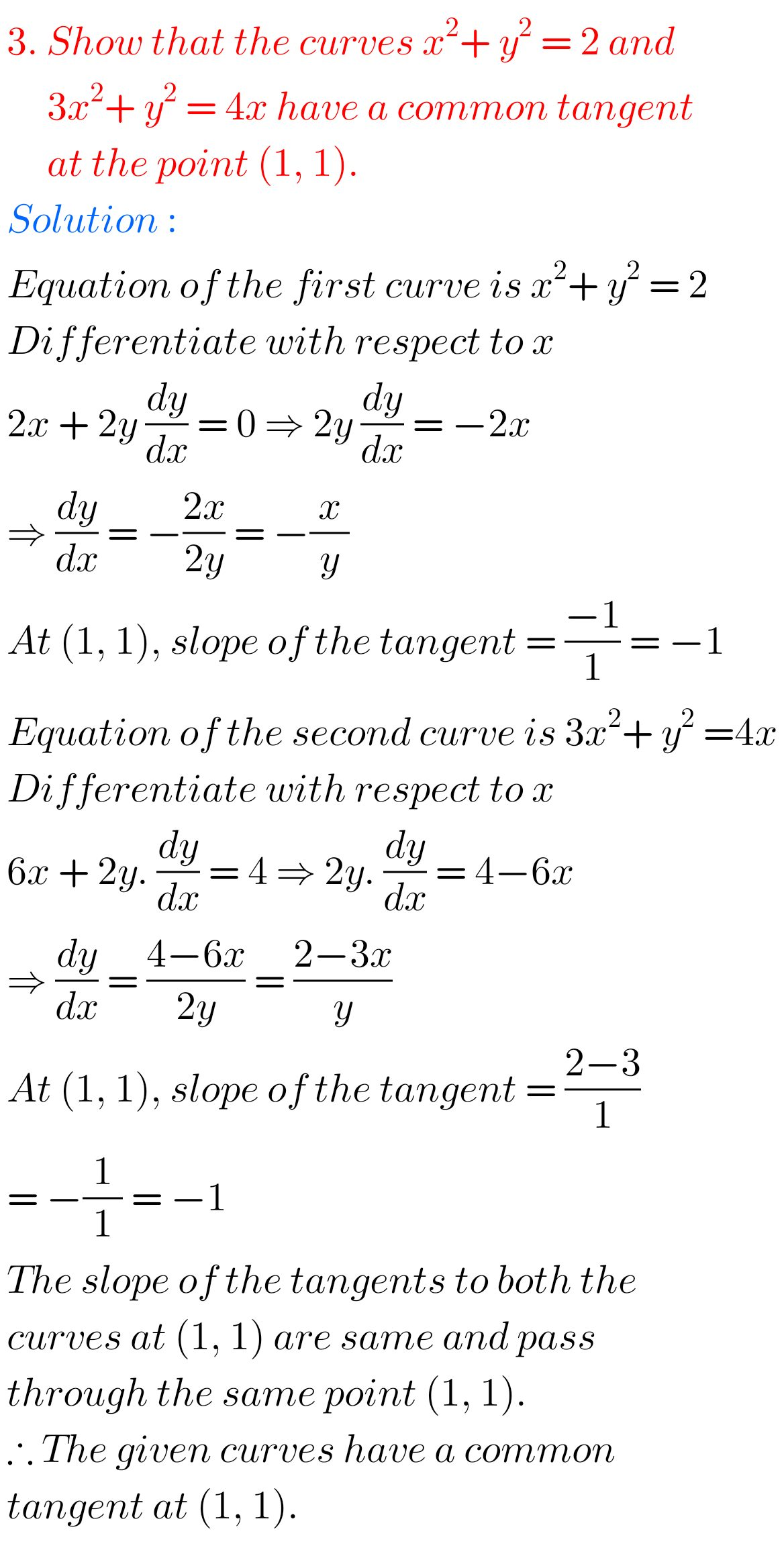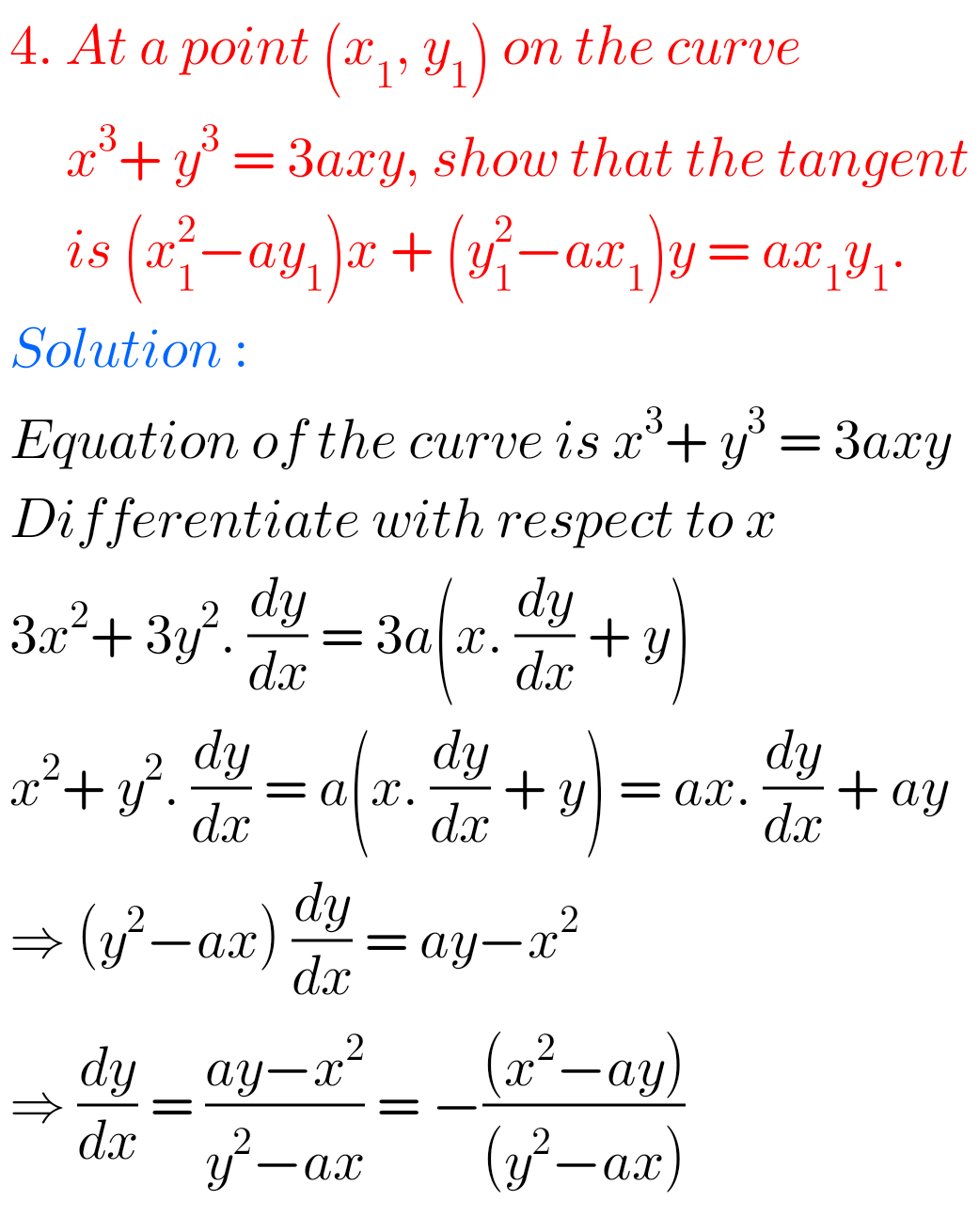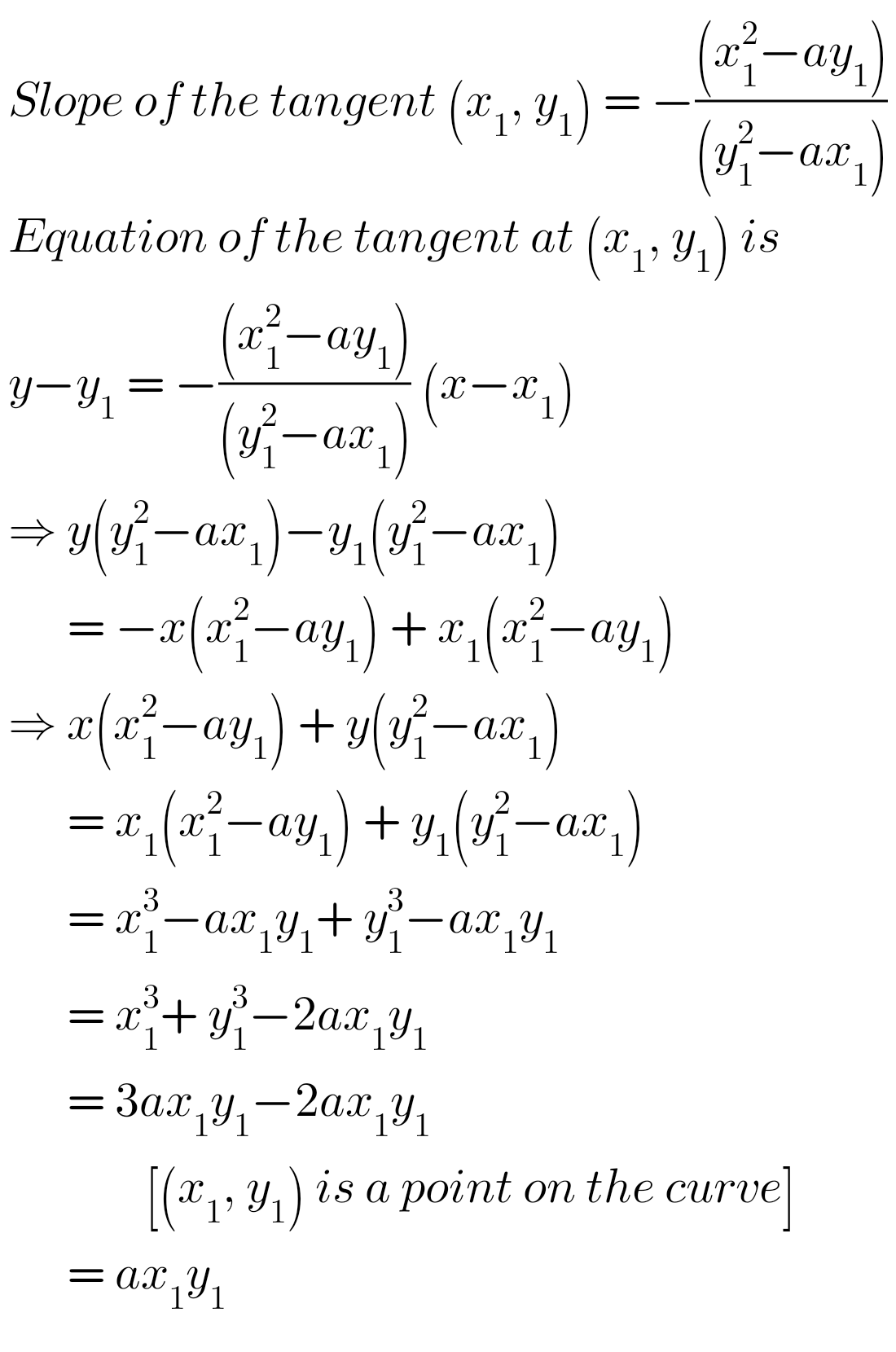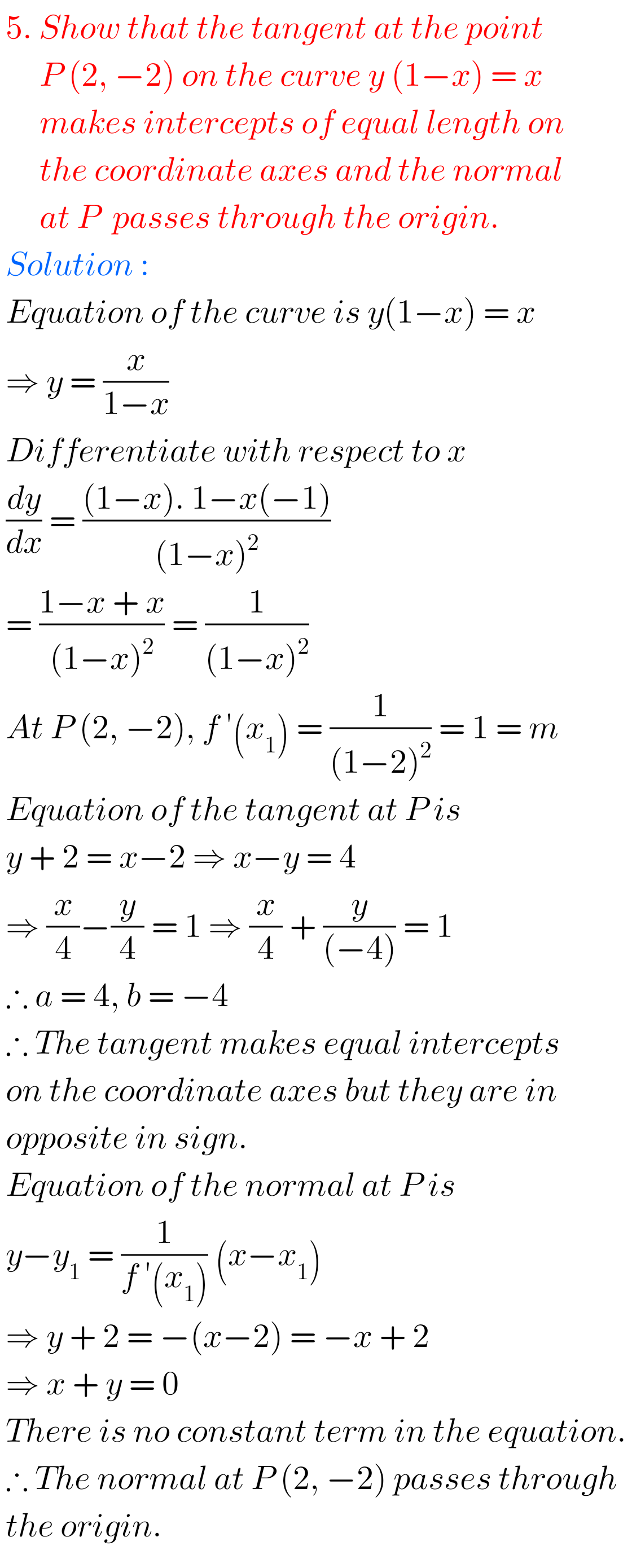FF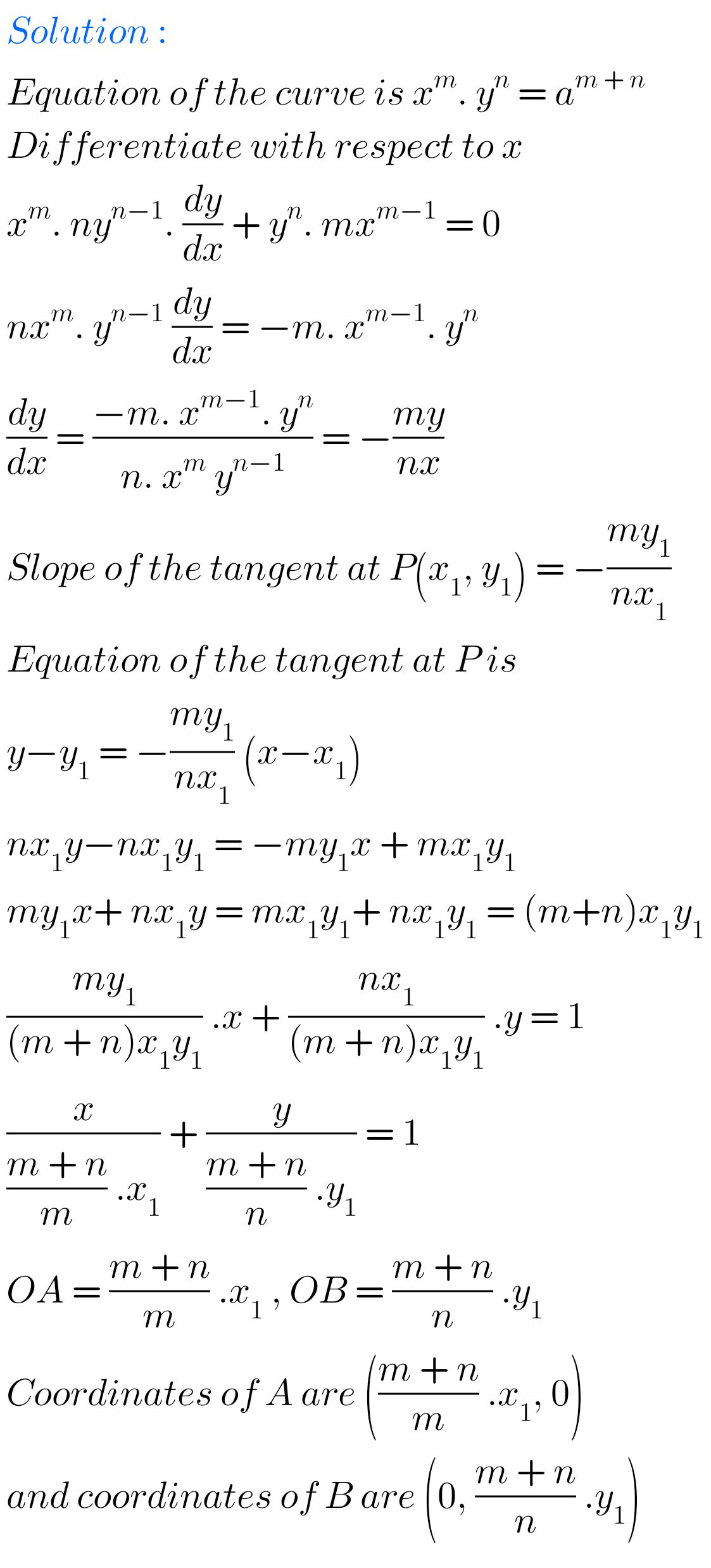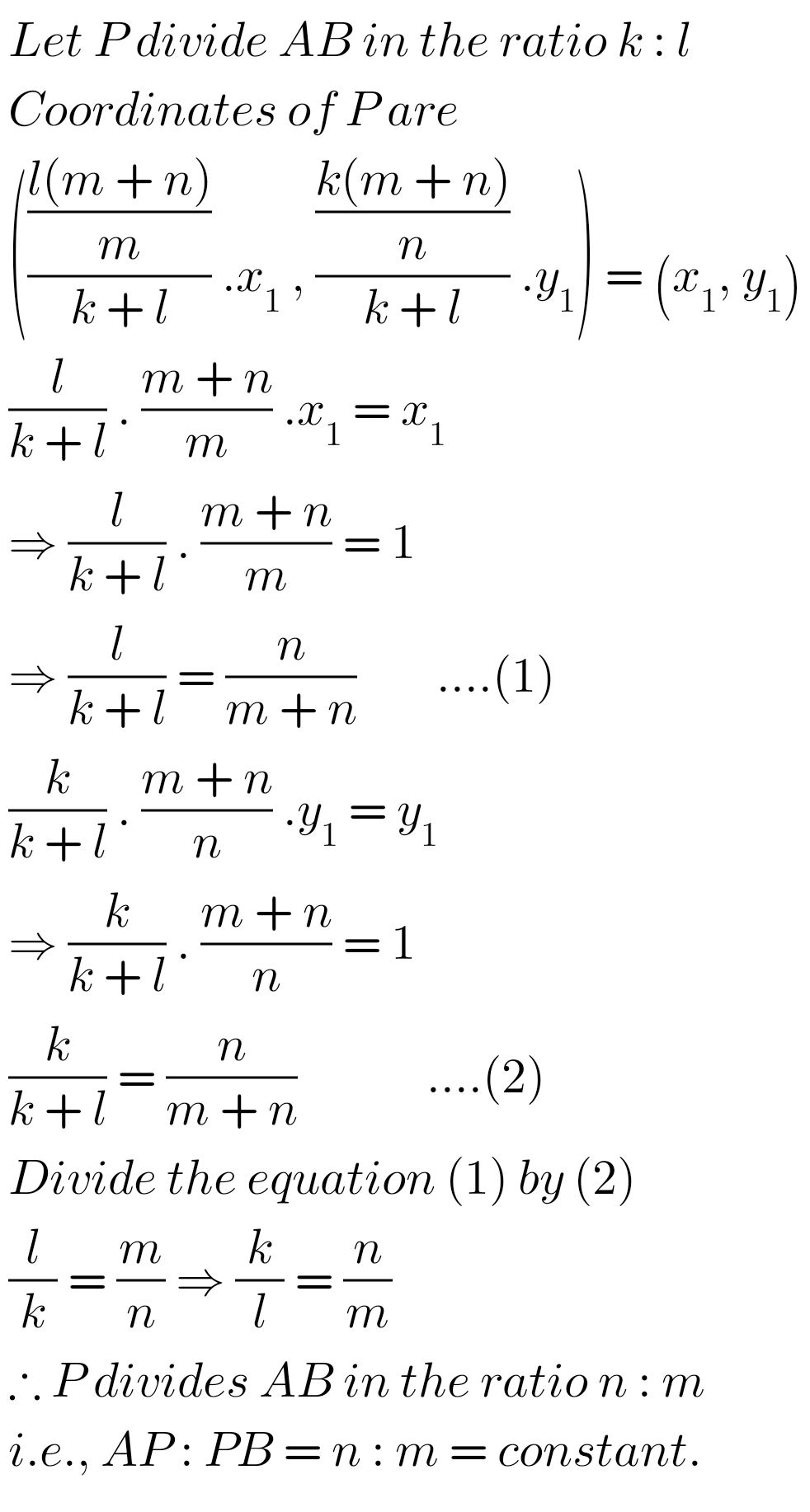Note : Observe the solutions and try them in your own method.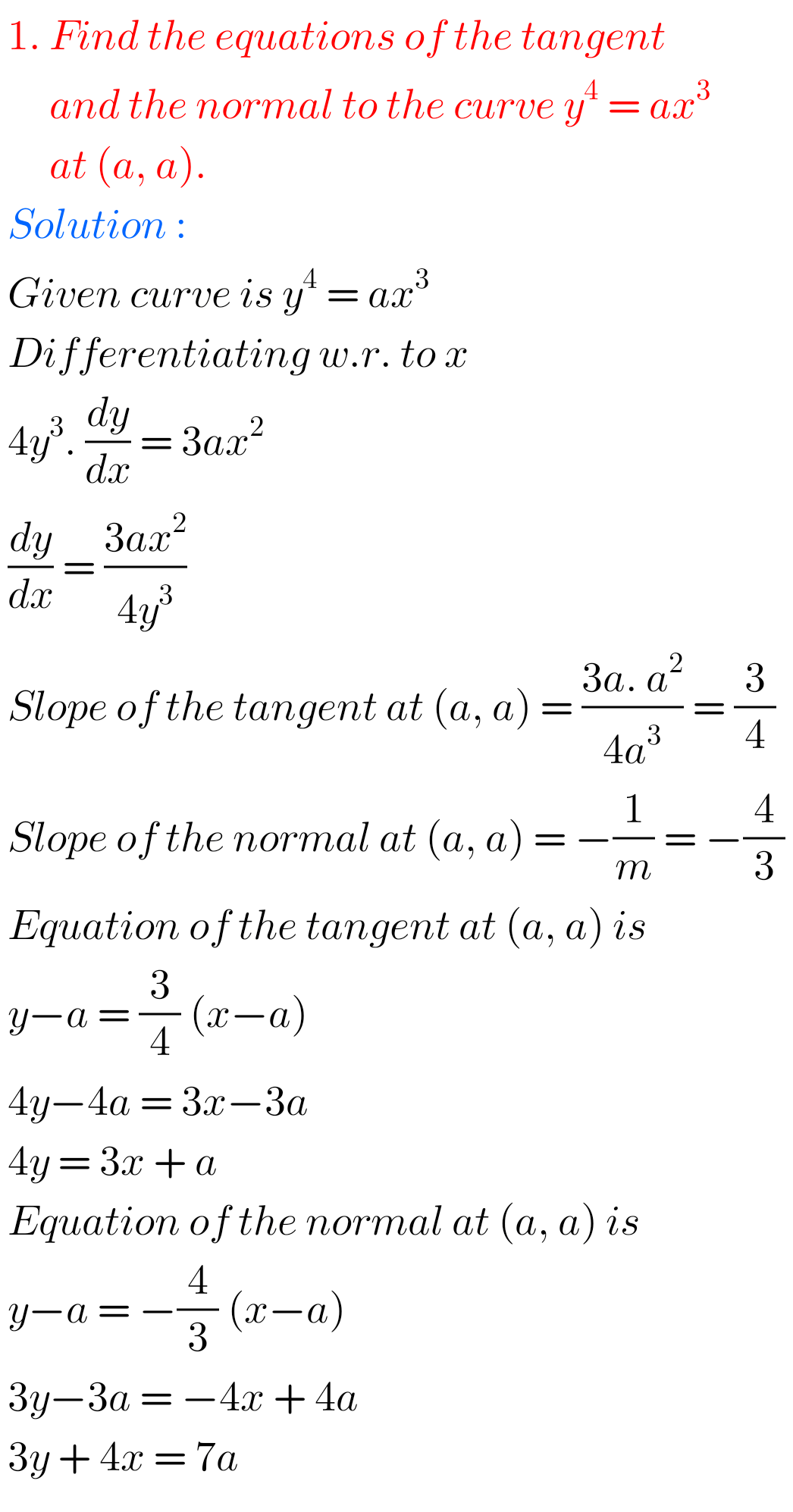SSC Maths solutions class 10